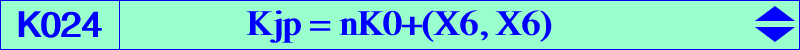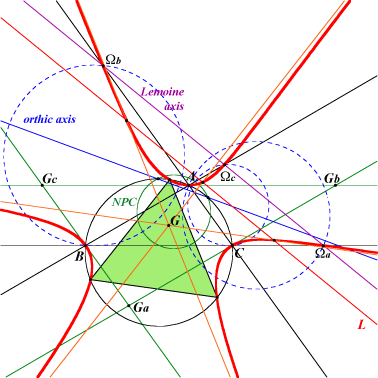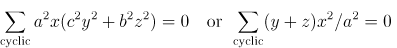Ωa, Ωb, Ωc : centers of the 3 Apollonian circles vertices of the (green) CircumTangential triangle anti-points, see Table 77 other points described below see other figures and a geometric construction belowKjp = K024 is the only isogonal nK0 which is a nK60+. Its root is the Lemoine point K. Kjp is a good example of stelloid. See the related stelloid K1136 and the bicircular quintic Q153, also K003 and Table 22. Kjp is mentioned in a paper by Shuzo TAKAMATSU and Shusaku OGINO : On Some Cubics connected with Simson lines- Tohoku Math. J. 43, p 99-103 (1937). K024 is a member of the classes CL004, CL022 and CL026 of cubics. The hessian cubic of Kjp is K193. The isotomic transform of Kjp is K196. Locus properties (see also Table 8) M* being the isogonal conjugate of M, and Ma, Mb, Mc the second intersections of the cevian lines of M with the circumcircle (i.e. the vertices of the circumcevian triangle of M), Kjp is the locus of point M such that : the circle with diameter MM* is orthogonal to the circumcircle. M and M* are conjugated with respect to the circumcircle i.e. M* lies on the polar line of M. This property is generalized in the page K687 and in CL022. See also the related cubics K085, K098, K105, K686. the pedal circle of M (and M*) is orthogonal to the nine-point circle. See two generalizations in Table 22 and Table 44. the center of the conic ABCMM* is collinear with M and M* (together with the union of the six bisectors). the pole of the line MM* in the conic ABCMM* is at infinity i.e. the tangents at M and M* to the conic ABCMM* are parallel. See also Z+(IK). the sum of line angles (BC,AM)+(CA,BM)+(AB,CM)=0 (mod. pi). Compare this with K003, property 4. See also a generalization in Table 22. the lines AM* and MbMc (or equivalently BM* and McMa, CM* and MaMb) are parallel. the parallels at Ma, Mb, Mc to the sidelines BC, CA, AB respectively are concurrent i.e. the triangles ABC and MaMbMc are parallelogic (together with the circumcircle, the line at infinity). One of the center of parallelogy is M* and the other lies on K409. the circumcevian triangles of M and M* are perspective (together with the union of the six bisectors, the circumcircle and the line at infinity). The locus of the perspector is K409. the pedal triangles of M and M* are parallelogic. See also CL022 and K150. See Table 8 for other parallelogic triangles. (more generally) Let t be a real number and P, Q two isogonal conjugates. PaPbPc, QaQbQc are the homothetic under h(P,t), h(Q,t) of the pedal triangles of P, Q respectively. PaPbPc and QaQbQc are parallelogic if and only if P (and Q) lie on Kjp (together with the line at infinity). With t = 1, we find property 10 again and with t = 2, these are the reflection triangles of P and Q. (more more generally) When P and Q are two isoconjugates under an isoconjugation with pole W, the locus is a member of CL022 (together with the line at infinity and the trilinear polar of the isotomic conjugate of the isogonal conjugate of W). the pedal and antipedal triangles of M are parallelogic (together with the circumcircle, the line at infinity). the pedal and circumcevian triangles of M are parallelogic (together with the circumcircle, the line at infinity). the antipedal and reflection triangles of M are parallelogic (together with the circumcircle, the line at infinity). the circumcevian and antipedal triangles of M are parallelogic (together with the circumcircle, the line at infinity). the circumcevian triangle of M and the triangle formed by the reflections of M about A, B, C (or about the sidelines of ABC) are parallelogic (together with the circumcircle, the line at infinity). Let P be a point. The parallels at A to PB, PC meet BC at Ab, Ac respectively and Oa is the circumcenter of AAbAc. Define Ob, Oc similarly. The triangles ABC and OaObOc are orthologic if and only if P lies on the union of Kjp and the Thomson cubic (from Hyacinthos #5618). In fact, Oa, Ob, Oc are collinear for any point P on Kjp. See also here (Angel Montesdeoca, 2018-01-13). The conic inscribed in ABC and in the circumcevian triangle of P is a parabola if and only if P lies on K024 (see comments here). See also K1065 for related topics. See also the related sextic Q169. Let P be a point with cevian triangle PaPbPc and circumcevian triangle ApBpCp. Using signed distances, the locus of P such that AAp / APa + BBp / BPb + CCp / CPc = 2 is K024, together with the line at infinity and the circumcircle (Francisco Javier Garcia Capitan, 2022-06-23). *** Locus properties related to Simson lines : Denote by sMa, sMb, sMc the Simson lines of Ma, Mb, Mc, and A1B1C1 the triangle bounded by these Simson lines (i.e. A1 = sMb /\ sMc, etc). sMa, sMb, sMc are concurrent if and only if M lies on Kjp (together with the circumcircle). This is the description given by Takamatsu and Ogino in the paper cited above. A1B1C1 and ABC are perspective if and only if M lies on Kjp (together with the circumcircle, the line at infinity, the cevian lines of O) A1B1C1 and ABC are parallelogic if and only if M lies on Kjp (together with the line at infinity). Notice that A1B1C1 and ABC are always orthologic. A1B1C1 and MaMbMc are parallelogic if and only if M lies on Kjp (together with the circumcircle, the line at infinity). Let P, P* be two isogonal conjugate points and A'B'C', A"B"C" the circumcevian triangles of P, P* resp. Denote sA', sB', sC' the Simson lines of A', B', C' wrt A"B"C" resp. These lines sA', sB', sC' are concurrent if and only if P lies on Kjp (together with the McCay cubic, the circumcircle). In other words, if P lies on Kjp then the lines sA', sB', sC' are concurrent (Hyacinthos #21685, Angel Montesdeoca).Points on the Kjp cubic The three real asymptotes are parallel to the sidelines of the Morley triangle and concur at G. Kjp intersects these asymptotes at A1, B1, C1 on the line L homothetic of the Lemoine axis under h(G,2/3). This is the satellite line of the line at infinity. It is also the trilinear polar of the isotomic conjugate of a^2(b^2+c^2)-8a^2c^2: : , the homothetic of X(76) under h(G,3/2). Thus, Kjp meets its asymptototes on a parallel to the trilinear polar of its root. This is true for any nK0(X6, P) with P on the Grebe cubic K102. Kjp passes through the centers of the three Apollonian circles, intersections of the Lemoine axis with the sidelines of ABC. The common tangentials of these points and the vertices of ABC are the intersections A0, B0, C0 of the sidelines of the antimedial triangle GaGbGc and the trilinear polar L' of X(308), isotomic conjugate of the Brocard midpoint X(39). L' is the satellite line of the Lemoine axis. Kjp also contains the vertices Ta, Tb, Tc of the circumtangential triangle (see TCCT, p.166, §6.28). These are the isogonal conjugates of the infinite points of the Morley sidelines. Hence, their tangentials are the points A1, B1, C1. Kjp obviously contains the isogonal conjugates A0*, B0*, C0* of the points A0, B0, C0 (on the perpendicular bisectors of ABC, on the lines through the vertices of ABC and the corresponding Apollonian centers, on a circumconic C' through X99) and A1, B1, C1 (on a circumconic C through X99, X1576, X1634). From them it is easy to derive several other points on the cubic. See the figure below.Kjp meets the three Apollonius circles again at nine points which are the vertices of three equilateral triangles having their sidelines perpendicular to the sidelines of the circumtangential triangle. These points lie on the cevian lines of the vertices Ta, Tb, Tc of the circumtangential triangle. They also lie three by three on two triads of lines passing through the isodynamic points and making 60° angles with one another. Obviously, Kjp contains the isogonal conjugates of these nine points. Note that the Kjp cubic and the McCay cubic meet the three Apollonius circles at the vertices of three regular hexagons. Actually, since Kjp is a stelloid, one can find infinitely many equilateral triangles inscribed in the curve.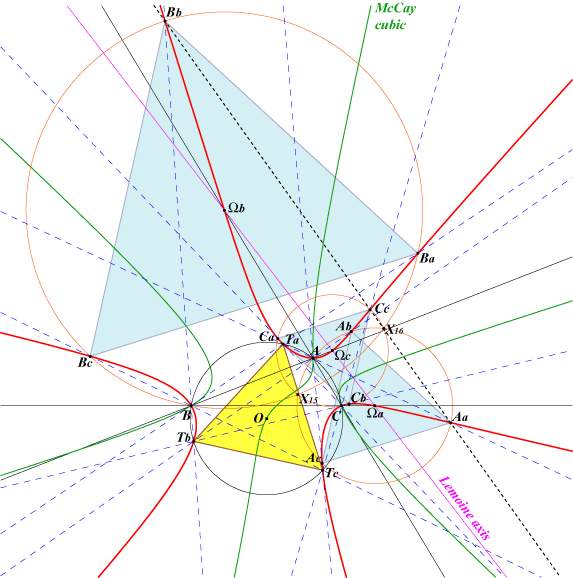Geometric construction of the Kjp cubic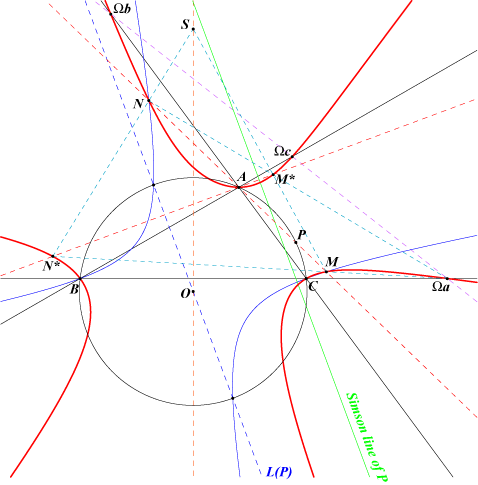Let P be a point on the circumcircle (O) of ABC and let L(P) be the parallel at O to the Simson line of P. L(P) meets (O) at two points. The line AP meets the rectangular hyperbola passing through these two points, B and C at M, N on the Kjp cubic. Denote by M*, N* the isogonal conjugates of M, N. Obviously, MN and M*N* meet at A. The lines MN* and M*N pass through the center of the A-Apollonian circle. The lines MM* and NN* meet at S on the perpendicular bisector of BC and on the tangent at P to (O). If L(P) is given, P is the isogonal conjugate of any perpendicular to L(P). Note that the perpendicular at A to the line AP intersects the same rectangular hyperbola at two points on the McCay cubic.Hessian and prehessians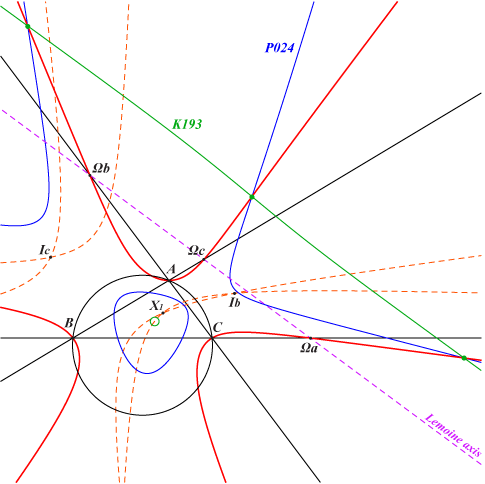The hessian of K024 is K193. K024 has three prehessians but one only P024 is real. This means that the hessian of P024 is K024. These three cubics generate a syzygetic pencil of cubics passing through the nine common inflexion points of K024. The prehessians have the same orthic line namely the Lemoine axis L. The polar conic of any point on L is a diagonal rectangular hyperbola passing through the in/excenters of ABC. With X(512) and X(669), we obtain the Stammler and Wallace hyperbolas represented on the figure (dashed orange curves). The polar conics of Ωa, Ωb, Ωc are the pairs of bisectors at A, B, C respectively. This gives a natural involution f on K024 that carries any point P to the center of the polar conic of P with respect to P024. See below for further properties of f. An equation of P024 is : ∑ b^2c^2(b^2 + c^2)x^3 = 3 ∏(c^2y + b^2 z)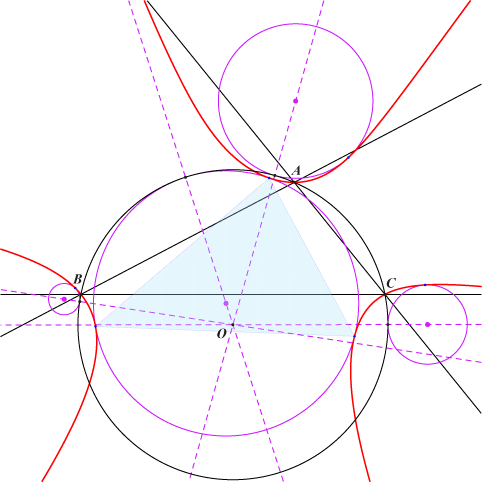The poloconic of any line ∆ with respect to P024 is a conic tritangent to K024. If ∆ meets K024 at three points, the points of tangency are their images under f. If ∆ is tangent to K024 at M, the conic is surosculating K024 at f(M) and is tangent at K024 at the image of the tangential of M. If ∆ is an inflexional tangent at M to K024, the conic has a sextuple contact with K024 and f(M) is said to be a sextactic point. There are four lines for which the conic is a circle and the corresponding conics are four circles tritangent to K024 and tangent to the circumcircle of ABC. This is the Feuerbach theorem for the cubic K024. The four circles are analogous to the in/excircles and the circumcircle is analogous to the nine point circle.The involution fThe definition above is useless if one wishes to construct the homologue M' of M. We shall consider that M' is the pole of M in a pencil of conics containing the Stammler rectangular hyperbola which is generated by the three conics (γA), (γB), (γC) defined as follows. All these conics are diagonal conics with respect to the CircumTangential triangle. An equation of (γA) is : 3a^2(c^2y^2 - b^2z^2) + 2(b^2 - c^2)(a^2 yz + b^2 zx + c^2 xy) = 0.(γA) passes through A and is tangent at this point to the circumcircle (O). It meets (O) again on the perpendicular bisector of BC that is on the bisectors at A in ABC. It meets AB, AC again at Ab, Ac on the line (δA) passing through the midpoint Ma of BC and the projection Ua of G on the parallel at A to BC. (γA) also contains the images of O and Oa under the homothety with center A and ratio 4/5. An easy computation shows that (γA) is in fact the polar conic in P024 of the infinite point of the line GΩa. Obviously, (γB), (γC) are defined similarly.Properties of f With P = u:v:w, the first coordinate of f(P) is : a^4 vw (∑ b^2c^2 u^2(v + w)) - a^2 vw (∑a^2 vw)(∑b^2c^2 u), the others being obtained cyclically. This clearly shows that f coincide with isogonal conjugation for any P on K024 and with X(32)-isoconjugation for any P on the circumcircle or the Lemoine axis. In all other cases, f(P) lies on the line passing through the X(6) and X(32)-isoconjugates of P which is the trilinear polar of the barycentric quotient X(110)÷M or equivalently the polar line of M in the Stammler hyperbola. This line contains the isogonal conjugate of P with respect to the CircumTangential triangle. The vertices T1, T2, T3 of the CircumTangential triangle are the singular points of f. The four common points of (γA), (γB), (γC) are the fixed points which also lie on the Stammler hyperbola (S) and on the cubics K729, K730. Recall that f globally fixes K024 and actually coincide with isogonal conjugation (in ABC) for any M on K024. More generally, if S is a point not lying on the sidelines of ABC, let ΩS be the pole of the isoconjugation that swaps S and f(S). Then, for any M on the cubic nK(ΩS, X6, S), f(M) is the ΩS-isoconjugate of M. Note that ΩS lies on the Brocard axis and that any such cubic nK contains T1, T2, T3. When S lies on the Lemoine axis or on the circumcircle, it must split into these two latter curves. For example, with S = X2, we obtain K734 = nK(X3, X6, X2). This involution f swaps • A, B, C and the centers of the Apollonius circles, • the centroid G and the circumcenter O of ABC, • the Lemoine axis and the circumcircle, • any line through G and a rectangular hyperbola through O and T1, T2, T3. Indeed, f maps G onto O which is the orthocenter of T1T2T3. These are the rectangular CircumTangential hyperbolas of Table 25. Note that M' = f(M) lies on the polar line L(M) of M in (S) which is also the trilinear polar of the barycentric quotient X(110)÷M. L(M) contains the Ω-isoconjugate of M wrt ABC where Ω is the barycentric square of any point on (S). In particular, L(M) contains the isogonal conjugate M* of M. Recall that (S) contains X(1), X(3), X(6), X(155), X(159), X(195), X(399), X(610), X(1498), X(1740), X(2574), X(2575), X(2916), X(2917), X(2918), X(2929), X(2930), X(2931), X(2935), X(2948), X(3216), etc. Hence f defines an isoconjugation with respect to the CircumTangential triangle and it is then easy to find many pivotal cubics in this triangle. We shall denote by pkT(P) the locus of M such that P, M, M' = f(M) are collinear. Naturally pkT(P) contains the seven singular/fixed points of f, also P (pivot) and P' (isopivot), the vertices of the cevian triangle of P wrt T1T2T3. The tangents at T1, T2, T3, P concur at P'. Moreover, pkT(P) meets (O) at the same points as pK(X32, P). The most remarkable cubics are the stelloid K726 = pkT(X6), K727 = pkT(X3), the circular cubic K728 = pkT(X23). Note that when P lies on the Euler line, it is always possible to find an isogonal pK, with pivot on GK, having the same points on (O) as pK(X32, P) and pkT(P).Parallels to the asymptotes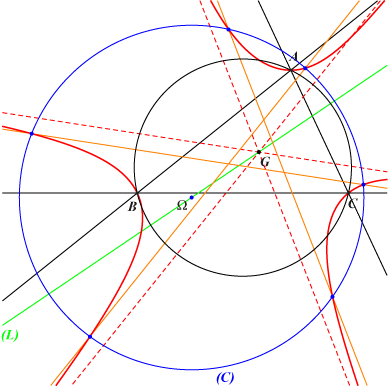Three parallels to the asymptotes of K024 forming a triangle with center G meet the cubic at six finite points lying on a same circle (C) with center Ω on the parallel (L) at G to the Brocard axis. On the other hand, three concurring parallels meet K024 at six finite points lying on a same rectangular hyperbola. See K003 for similar properties.